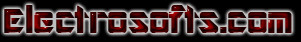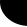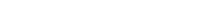HOME Electronics Directory Articles/ Tutorials eBooks About Us FORUM Links Contact UsSystemVerilog Tutorial PART IV: by Abhiram Rao

Control Statements in SystemVerilog

<Previous    Page       Next>

Wait, what's this? if, else, repeat, while, for, case - it's Verilog that looks exactly like C (and probably whatever other language you're used to programming in)! Even though the functionality appears to be the same as C, Verilog is an HDL, so the descriptions should translate to hardware. This means you've got to be careful when using control statements (otherwise your designs might not be implementable in hardware).

if-else

if-else statements check a condition to decide whether or not to execute a portion of code. If a condition is satisfied, the code is executed. Else, it runs this other portion of code.

 ` // begin and end act like curly braces in C/C++.` `if (enable == 1'b1)` ` begin` `  data = 10; // Decimal assigned` `  address = 16'hDEAD; // Hexadecimal` `enable = 1'b1; // Binary  ` `end ` `else ` `begin` `  data = 32'b0;` `   enable = 1'b0;` `  address = address + 1;  ` `end `

One could use any operators in the condition checking as in the case of C language. If needed we can have nested if else statements, statements without else is also ok, but then it has its own problem when modeling combinational logic, if statement without else results in a Latch (this is not always true).

case

Case statements are used where we have one variable which needs to be checked for multiple values. Like an address decoder, where the input is an address and it needs to check for all the values that it can take. Instead of using multiple nested if-else statements, one for each value we're looking for, we use a single case statement, and this is similar to switch statements in languages like C++.

Case statements begin with the reserved word case and end with the reserved word endcase (Verilog does not use brackets to delimit blocks of code). The cases, followed with a colon and the statements you wish executed, are listed within these two delimiters. It's also a good idea to have a default case. Just like with a finite state machine (FSM), if the Verilog machine enters into a non-covered statement, the machine hangs. Defaulting the statement with a return to idle keeps us safe.

 ` case (address)` ` 0: \$display ("It is 4:00 PM");` ` 1: \$display ("I am feeling sick");` ` 2: \$display ("Time to have a coffee");` ` default : \$display ("Need to complete");` `endcase`

Looks like address value was 3 and so I am still writing this tutorial. Note: One thing that is common to if-else and case statement is that, if you don't cover all the cases (don’t have else in if-else or default in case), and you are trying to write a combination statement, the synthesis tool will infer Latch.

While

A while statement executes the code within it repeatedly if the condition it is assigned to check returns true. While loops are not normally used for models in real life, but they are used in test benches. As with other statement blocks, they are delimited by begin and end.

 ` while(free) ` ` begin` `     \$display ("Continue with the webpage ");` ` end`

As long as free variable is set, code within the begin and end will be executed. i.e. print "Continue with web development". Lets look at a stranger example, which uses most of the constructs of Verilog. Well you heard it right. Verilog has very few reserve words then VHDL, and in this few, we use even lesser few for actual coding.

 `module counter (clk,rst,enable,count);` `input clk, rst, enable;` `output [3:0] count;` `reg [3:0] count;` `                                  ` `always @ (posedge clk or posedge rst)` `if (rst) begin` `  count <= 0;` `end else begin : COUNT` `  while (enable) begin` `    count <= count + 1;` `    disable COUNT;` `  end` `end` `endmodule`
` `
` `

The example above uses most of the constructs of Verilog. You'll notice a new block called always - this illustrates one of the key features of Verilog. Most software languages, as we mentioned before, execute sequentially - that is, statement by statement. Verilog programs, on the other hand, often have many statements executing in parallel. All blocks marked always will run - simultaneously - when one or more of the conditions listed within it is fulfilled.

In the example above, the always block will run when either rst or clk reaches a positive edge - that is, when their value has risen from 0 to 1. You can have two or more always blocks in a program going at the same time (not shown here, but commonly used).

We can disable a block of code, by using the reserve word disable. In the above example, after each incrementation of the counter, the COUNT block of code (not shown here) is disabled.

for loop

For loops in Verilog are almost exactly like for loops in C or C++. The only difference is that the ++ and -- operators are not supported in Verilog. Instead of writing i++ as you would in C, you need to write out its full operational equivalent, i = i + 1.

 `   for (i = 0; i < 16; i = i +1) ` `  begin` `     \$display ("Current value of i is %d", i);` `  end `

This code will print the numbers from 0 to 15 in order. Be careful when using for loops for register transfer logic (RTL) and make sure your code is actually sanely implementable in hardware.. and that your loop is not infinite.

repeat

Repeat is similar to the for loop we just covered. Instead of explicitly specifying a variable and incrementing it when we declare the for loop, we tell the program how many times to run through the code, and no variables are incremented (unless we want them to be, like in this example).

 `repeat (16)` `begin` `    \$display ("Current value of i is %d", i);` `     i = i + 1;` `end `

The output is exactly the same as the previous for-loop program example. It is relatively rare to use a repeat (or for-loop) in actual hardware implementation.

Summary

• while, if-else, case(switch) statements are same as C language.
• An if-else and case statement requires all the cases to covered for combinational logic.
• for-loop same as C, but no ++ and -- operators.
• repeat is the same as for-loop but without the incrementing variable.

Variable Assignment

In digital there are two types of elements, combinational and sequential. Of course we know this. But the question is "how do we model this in Verilog". Well Verilog provides two ways to model the combinational logic and only one way to model sequential logic.

• Combination elements can be modeled using assign and always statements.
• Sequential elements can be modeled using only always statement.
• There is third type, which is used in test benches only, it is called initial statement.

Initial Blocks

Initial block as name suggests, is executed only once and that too, when simulation starts. This is useful in writing test bench. If we have multiple initial blocks, then all of them are executed at beginning of simulation.

Example

 `initial ` `begin` `    clk = 0;` `    reset = 0;` `    req_0 = 0;` `  req_1 = 0;` `end`In the above example at the beginning of simulation, (i.e when time = 0), all the variables inside the begin and end are driven zero.

Always Blocks

As name suggest, always block executes always. Unlike initial block, which executes only once, at the beginning of simulation? Second difference is always block should have sensitive list or delay associated with it.

Sensitive list is the one which tells the always block when to execute the block of code, as shown in figure below. @ Symbol after the always reserved word indicates that always block will be triggers "at" condition in parenthesis after symbol @.

One important note about always block is, it can not drive a wire data type, but can drive reg and integer data type.

 `always @ (a or b or sel)` `begin` `  y = 0;` `  if (sel == 0) ` ` begin` `    y = a;` `  end ` ` else ` ` begin` `    y = b;` `  end` `end`

Above example is a 2:1 mux, with input a and b, sel is the select input and y is mux output. In any combination logic output is changes, whenever the input changes. This theory when applied to always blocks means that, the code inside always block needs to be executed when ever the input variables (or output controlling variables) change. This variables are the one which are included in the sensitive list, namely a, b and sel.

There are two types of sensitive list, the one which are level sensitive ( like combinational circuits) and the one which are edge sensitive (like flip-flops). Below the code is same 2:1 Mux but the output y now is output of a flip-flop.

` `
 `always @ (posedge clk)` `begin` ` if (reset == 0) ` ` begin` `  y <= 0;` ` end ` ` else if (sel == 0) ` ` begin` `  y <= a;` ` end ` ` else ` ` begin` `  y <= b;` ` end` `end`

We normally have reset to flip-flops, thus every time clock makes transition from 0 to 1 (posedge), we check if reset is asserted (synchronous reset), and followed by normal logic. If we look closely we see that in the case of combinational logic we had "=" for assignment, and for the sequential block we had "<=" operator. Well "=" is blocking assignment and "<=" is nonblocking assignment. "=" executes code sequentially inside a begin and end, where as nonblocking "<=" executes in parallel. We can have always block without sensitive list, in that case we need to have delay as shown in code below.

 always begin  #5 clk = ~clk; `end`

#5 in front of the statement delays the execution of the statement by 5 time units.

Assign Statement

Assign statement is used for modeling only combinational logic and it is executed continuously. So assign statement called continuous assignment statement as there is no sensitive list.

`assign out = (enable) ? data : 1'bz;`

Above example is a tri-state buffer. When enable is 1, data is driven to out, else out is pulled to high-impedance. We can have nested conditional operator to construct mux, decoders and encoders.

`assign out = data;`

Above example is a simple buffer.

<Previous    Page       Next>